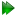Subsections

Free rotor isotropic cone equations

Figure 16.11: The free rotor isotropic cone model simulated and calculated in-frame Daeg(1) and Daeg(2) frame order matrix elements. The top row corresponds to Daeg(1) and the bottom to Daeg(2). In these plots, θX corresponds to the cone opening half-angle θ. Frame order matrix values have been calculated every 10 degrees.Figure 16.12: The free rotor isotropic cone model simulated and calculated out-of-frame Daeg(1) and Daeg(2) frame order matrix elements. The top row corresponds to Daeg(1) and the bottom to Daeg(2). In these plots, θX corresponds to the cone opening half-angle θ. Frame order matrix values have been calculated every 10 degrees.Free rotor isotropic cone rotation matrices

The rotation matrix is the full torsion-tilt rotation matrix of equation 12.74c on page.

Free rotor isotropic cone frame order matrix

The frame order matrix isThe surface normalisation factor isFree rotor isotropic cone 1 degree frame order

The 1 degree frame order matrix with tensor rank-2 isFree rotor isotropic cone 2 degree frame order

The 2 degree frame order matrix with tensor rank-4 consists of the following elements, using Kronecker product double indices from 0 to 8Free rotor isotropic cone frame order matrix simulation and calculation

The frame order matrix element simulation script from Section 16.2, pagewas used to compare the implementation of equations 16.36 and 16.37 above. Frame order matrix Daeg(1) and Daeg(2) values were both simulated and calculated, both within and out of the motional eigenframe. The in-frame Daeg(1) and Daeg(2) values are shown in figure 16.11. The out-of-frame Daeg(1) and Daeg(2) values are shown in figure 16.12.

The relax user manual (PDF), created 2020-08-26.